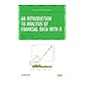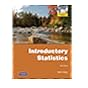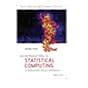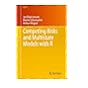Dr. S. R. Lasker Library Online Catalogue

Normal view

# An introduction to statistical computing : a simulation-based approach / Jochen Voss

Material type:TextLanguage: English Publication details: 2014. Description: xii, 382 pages : illustrations ; 24 cmISBN: 9781118357729 (hardback); 1118357728 (hardback)DDC classification: 519.50113 LOC classification: QA276.4 | .V66 2014Online resources: WorldCat details | E-book Fulltext
Contents:
TOC Pseudo random number generators -- 1.1.1. The linear congruential generator -- 1.1.2. Quality of pseudo random number generators -- 1.1.3. Pseudo random number generators in practice -- 1.2. Discrete distributions -- 1.3. The inverse transform method -- 1.4. Rejection sampling -- 1.4.1. Basic rejection sampling -- 1.4.2. Envelope rejection sampling -- 1.4.3. Conditional distributions -- 1.4.4. Geometric interpretation -- 1.5. Transformation of random variables -- 1.6. Special-purpose methods -- 1.7. Summary and further reading -- Exercises -- 2. Simulating statistical models -- 2.1. Multivariate normal distributions -- 2.2. Hierarchical models -- 2.3. Markov chains -- 2.3.1. Discrete state space -- 2.3.2. Continuous state space -- 2.4. Poisson processes -- 2.5. Summary and further reading -- Exercises -- 3. Monte Carlo methods -- 3.1. Studying models via simulation -- 3.2. Monte Carlo estimates -- 3.2.1.Computing Monte Carlo estimates.
Summary: "This is a book about exploring random systems using computer simulation and thus, this book combines two different topic areas which have always fascinated me: the mathematical theory of probability and the art of programming computers"--
Tags from this library: No tags from this library for this title.
Star ratingsAverage rating: 0.0 (0 votes)
Holdings
Item type Current library Collection Call number Copy number Status Date due Barcode Item holdsE-Book
E-book
Non-fiction 519.50113 VOI 2014 (Browse shelf(Opens below)) Not for loanText
Reserve Section
Non-fiction 519.50113 VOI 2014 (Browse shelf(Opens below)) C-1 Not For Loan 27381Text
Circulation Section
Non-fiction 519.50113 VOI 2014 (Browse shelf(Opens below)) C-2 Available 27382
Total holds: 0
##### Browsing EWU Library shelves, Shelving location: Circulation Section Close shelf browser (Hides shelf browser)519.5 TSI 2013 An introduction to analysis of financial data with R / 519.5 WEI 2012 Introductory statistics. 519.5 WEI 2012 Introductory statistics. 519.50113 VOI 2014 An introduction to statistical computing : 519.502 BEC 2012 Competing risks and multistate models with R / 519.502 BEC 2012 Competing risks and multistate models with R / 519.502 BEC 2012 Competing risks and multistate models with R /

Includes bibliographical references (pages 375-377) and index.

TOC Pseudo random number generators --
1.1.1. The linear congruential generator --
1.1.2. Quality of pseudo random number generators --
1.1.3. Pseudo random number generators in practice --
1.2. Discrete distributions --
1.3. The inverse transform method --
1.4. Rejection sampling --
1.4.1. Basic rejection sampling --
1.4.2. Envelope rejection sampling --
1.4.3. Conditional distributions --
1.4.4. Geometric interpretation --
1.5. Transformation of random variables --
1.6. Special-purpose methods --
1.7. Summary and further reading --
Exercises --
2. Simulating statistical models --
2.1. Multivariate normal distributions --
2.2. Hierarchical models --
2.3. Markov chains --
2.3.1. Discrete state space --
2.3.2. Continuous state space --
2.4. Poisson processes --
2.5. Summary and further reading --
Exercises --
3. Monte Carlo methods --
3.1. Studying models via simulation --
3.2. Monte Carlo estimates --
3.2.1.Computing Monte Carlo estimates.

"This is a book about exploring random systems using computer simulation and thus, this book combines two different topic areas which have always fascinated me: the mathematical theory of probability and the art of programming computers"--

AS

Sagar Shahanawaz

There are no comments on this title.